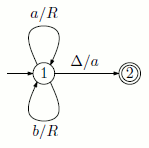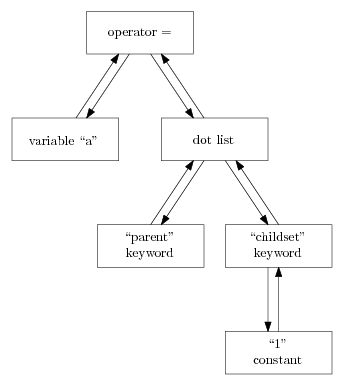## pcosmos.ca

### Philippe Choquette's universe

 PCASTL ExamplesGenetic Programming This example comes from section 10.2 (Symbolic Regression with Constant Creation) of the book Genetic Programming: on the programming of computers by means of natural selection of John R. Koza, ISBN 978-0-262-11170-6.The curve to discover is: 2.718x2 + 3.1416x The file sym-reg-test-cases.dat contains 20 random values of x between -1 and 1. The second column contains the ordinates of the curve. The used program is gp-example.astl. See comments inside to run it on your computer. On Windows, the solution found at generation 44 is: x * (x + (-0.2162846767 - x + 0.7376628925 + (pdiv(x, 0.9688711203) + (pdiv(x, 0.8065126499) - (-0.03921628468 - x + (x + 0.01388592181)) + (-0.4843592639 + 0.9697866756) * x + 0.7376628925) + -0.03921628468 * x + 0.4240546892)) + (x + x * -0.8399609363) * x * (x - x) * pdiv(x, pdiv(0.9697866756, 0.9688711203) + x * (x - 0.9697866756 * (x + x * x - x * x))) + 0.4240546892) + x Expression which is equivalent to: 2.71825x2 + 3.13248x On Linux the convergence is faster. On Mac OS, two additional trials with different arguments to srand were necessary to me. A solution was found with 1979 as seed instead of 42.Iterative Code Generation The function make_trans_func used in the examples below eliminates repetitive statements. Without it in the pushdown automaton example, the code would look like: ```# Transitions (non self-modifying) make_trans = function(init_state, input_symbol, popped_symbol, new_state, pushed_symbol) { transition = names("init_state", "input_symbol", "popped_symbol", "new_state", "pushed_symbol") transition.init_state = init_state transition.input_symbol = input_symbol transition.popped_symbol = popped_symbol transition.new_state = new_state transition.pushed_symbol = pushed_symbol transition } ```Turing Machines Turing machine #1. Require gen-trans-func.astl and run_machine.astl.Near transitions, the two symbols (x / y) are: x: the symbol under the head y: the action taken The action can be: R: move the head to the right L: move the head to the left other: the symbol to write The empty space is represented by Delta. Turing machine #2. Require gen-trans-func.astl and run_machine.astl.Pushdown Automaton A pushdown automaton. Require gen-trans-func.astl.Over transitions, the three symbols (x, y; z) are: x: the symbol read y: the symbol popped z: the symbol pushed The empty sequence is represented by lambda.Finite AutomatonThe following code demonstrates one of three ways of defining a function that alternatively displays two results. ```# first way # function definition: a = function() { print(1) if (value(a.childset.childset.childset.childset) == 1) { a.childset.childset = `print(2)' } else { a.childset.childset = `print(1)' } {} } # function calls a() a() ``` In the first way: a.childset would give a reference to the parameter name list of the "a" function. The function definition tree structure is illustrated here. a.childset gives a reference to the statement list of the "a" function. a.childset.childset gives a reference to the first statement in the previous list. a.childset.childset.childset would give a reference to function name node of the "print" call. The function call tree structure is illustrated here. a.childset.childset.childset gives a reference to the argument list of the "print" call. a.childset.childset.childset.childset gives a reference to the first element of the previous list, ie to the first argument of the "print" call. One line comments have to begin with # and we end the statement list of the "a" body with {} to prevent the display of the value of the assignation in the "else". ```# second way a = function() { print(1) # arglist, value call, operator==, if, stmtlist if (value(parent.parent.parent.parent.parent .childset.childset.childset) == 1) { a.childset.childset = `print(2)' } else { a.childset.childset = `print(1)' } {} } a() a() ``` In the second way: parent gives a reference to the argument list node of the "value" call. parent.parent gives a reference to the "value" call node. parent.parent.parent gives a reference to the operator == node. This node is also the condition node of the "if" statement. parent.parent.parent.parent gives a reference to the "if" node. parent.parent.parent.parent.parent gives a reference to the statement list node of the "a" function. The additional .childset gives a reference to the "print" call node. The additional .childset gives a reference to the argument list of the "print" call. The additional .childset gives a reference to the first argument of the "print" call. ```# third way a = function() { print(1) b = parent.parent.childset.childset.childset if (value(b) == 1) { *b = `2' } else { *b = `1' } {} } a() a() ``` In the third way, we use the unary operator * meaning that we want the assignation to be done to the reference holded by the variable "b". If they were not inside a function body, the "else" could not be placed at the beginning of a new line. Placing an "else" at the beginning of a new line would result in a syntax error. A function displaying alternatively three results. A function generating functions displaying alternatively n results.Code Segments You can use code segments as starting points for genealogical dotted lists. ```> info(` "abc" ') [node_type] "code segment" [nb_children] 1 > > info(` "abc" '.childset) [node_type] "string" [nb_children] 0 [value] "abc" > > info(` "abc" != "cba" '.childset) [node_type] "relational or logical operator" [nb_children] 2 [operator] "!=" > > info(` function(x) x '.childset) [node_type] "function definition" [nb_children] 2 [parameters] "x" ``` When you assign a code segment to an existing node, you do not have to get the code root using the `{code here}'.childset form. Using the `{code here}' form is enough.Other Examples A recursive function. Basic Examples The following session example demonstrates the use of the keywords and the use of the info internal function which gives the type of the node reference it receives as argument and some valuable information about the node. PCASTL statements are after the '>' symbols and interpreter output is the text on the lines beginning with spaces. ```> a = parent 0x4318e0 > info(a) [node_type] "mathematical operator" [nb_children] 2 [operator] = > > info(a.childset) [node_type] "variable" [nb_children] 0 [name] "a" > > info(a.childset) [node_type] "list" [nb_children] 1 > > info(a.childset.childset) [node_type] "parent reserved word" [nb_children] 0 > > a.childset.parent 0x4318e0 > a.childset.parent 0x4318e0 ``` After the assignation of a value to a variable, the interpreter returns the value. The address we see (0x330878) is in this case the address of the node containing the operator. We also get an address when we define a function and when we declare an explicit code segment. The code we just used is illustrated in the image below.A genealogical dotted list is a list of "parent" and "childset" reserved words separated by dots. The "parent" reserved word alone is a genealogical dotted list of one item. The following session example uses a genealogical dotted list of two items. ```> a = parent.childset 0x431560 > info(a) [node_type] "list" [nb_children] 2 > > info(a.childset) [node_type] "childset reserved word" [nb_children] 1 > > info(a.childset.childset) [node_type] "numerical constant" [nb_children] 0 [value] 1 ``` The structure of the first statement in the previous example is illustrated below.PCASTL on Wikipedia (with very basic examples).   back to PCASTL